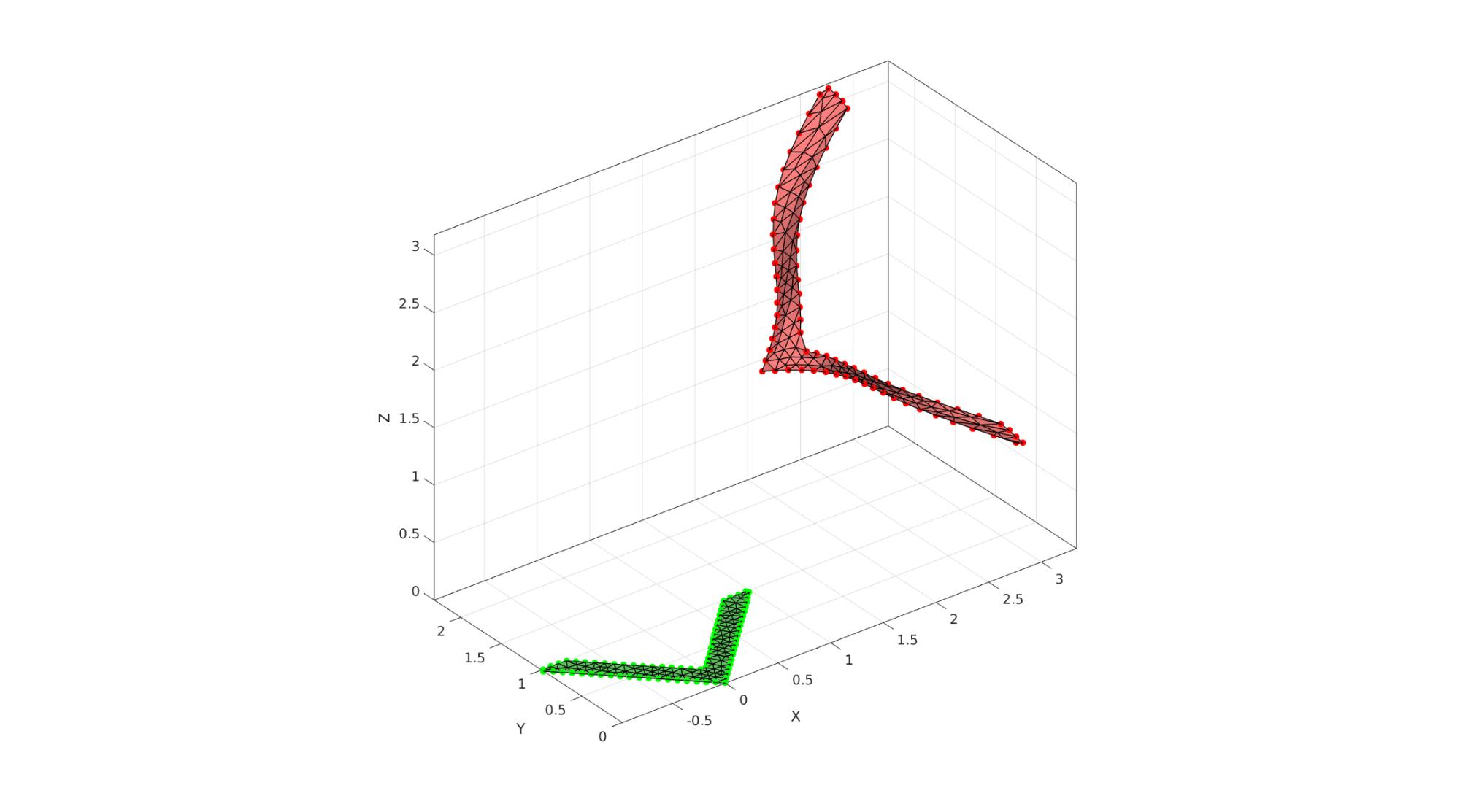# meanValueCoordinates

Below is a demonstration of the features of the meanValueCoordinates function

## Contents

```clear; close all; clc;
```

## Syntax

[C]=meanValueCoordinates(V_cage,V_interp);

Work in progress

## Examples

Plot settings

```lineWidth = 1;
markerSize = 25;
```

## Example 1:

Example template cage points

```Vc = [0 0; 1 1; 0.75 1; 0 0.25; -0.75 1; -1 1;];
V_cage_template=evenlySampleCurve(Vc,75,'linear',1);
V_cage_template(:,3)=0;

% Create template mesh
[F_mesh,V_mesh]=regionTriMesh2D({V_cage_template(:,[1 2])},[],0,0);
V_mesh(:,3)=0;
```

## Create target cage points

```% Final position of the cage.
V_cage_target=V_cage_template;
V_cage_target(:,2)=V_cage_target(:,2);
t=V_cage_target(:,2); t=t-min(t); t=t./max(t);
V_cage_target(V_cage_target(:,1)>0,1)=V_cage_target(V_cage_target(:,1)>0,1)+(t(V_cage_target(:,1)>0)).^2;
V_cage_target(V_cage_target(:,1)<0,2)=V_cage_target(V_cage_target(:,1)<0,2)+(t(V_cage_target(:,1)<0)).^2;
V_cage_target(:,3)=0.25*sin(t*2*pi);

R=euler2DCM([-0.25*pi -0.25*pi 0.25*pi]);
V_cage_target=V_cage_target*R;
V_cage_target=V_cage_target+1.5;
```
```[C]=meanValueCoordinates(V_cage_template,V_mesh);

V_mesh_warp=C*V_cage_target;
```
```cFigure; hold on;
plotV(V_cage_template, 'g.-','LineWidth', lineWidth, 'MarkerSize', markerSize);
gpatch(F_mesh,V_mesh,'gw','k',1,lineWidth)

plotV(V_cage_target, 'r.','LineWidth', lineWidth, 'MarkerSize', markerSize);
gpatch(F_mesh,V_mesh_warp,'rw','k',1,lineWidth)

drawnow;
```GIBBON www.gibboncode.org

Kevin Mattheus Moerman, [email protected]

GIBBON footer text

GIBBON: The Geometry and Image-based Bioengineering add-On. A toolbox for image segmentation, image-based modeling, meshing, and finite element analysis.

Copyright (C) 2006-2023 Kevin Mattheus Moerman and the GIBBON contributors

This program is free software: you can redistribute it and/or modify it under the terms of the GNU General Public License as published by the Free Software Foundation, either version 3 of the License, or (at your option) any later version.

This program is distributed in the hope that it will be useful, but WITHOUT ANY WARRANTY; without even the implied warranty of MERCHANTABILITY or FITNESS FOR A PARTICULAR PURPOSE. See the GNU General Public License for more details.

You should have received a copy of the GNU General Public License along with this program. If not, see http://www.gnu.org/licenses/.